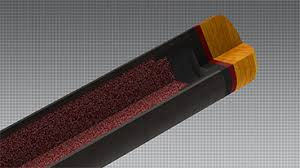top of page
Search

# Choosing the right break shaft

Updated: Jan 30, 2019

Heavy? Light? Phenolic tip? Oh what to do? Hopefully we have some answers...There are so many opinions about what you want in a break cue.

Light is Right!

I understand the physics of mass times velocity to generate cue ball momentum, but which is more important?

As it turns out, it is easier to create power by lightening the cue and increasing velocity, but there is a point of diminishing return. I have seen everything from 17 to 28 oz in a breaking cue. Physics tells us that we want the cue moving fast, and minimal energy lost through a flexing or compressing cue.

Break cue weight preference should be based on simple physics. Here's the "simple" physics:

The momentum of the cue ball is what matters, relative to the rack. The equation for Momentum is Mass X Velocity. The mass here isn't the mass of the stick, it's the mass of the CB. That mass is always around 6 oz, it isn't going to change. Therefore, velocity is the only factor we need to take into account. All we have to worry about is propelling the CB as fast as possible (while still maintaining control, of course). This is known as Impulse. Impulse is Force X Time. Force is Mass X Acceleration. Time is contact time between cue stick and cue ball. This mass is the mass of the cue stick, acceleration is the change in velocity of the cue stick from rest to contact.

Momentum of CB = Mass of CB X Velocity of CB    Impulse of CB = Force generated by cue stick X Contact Time    Force generated by cue stick = Mass of cue stick X Acceleration of cue stick

Intuitively, it looks as though, since mass (of the stick) and acceleration have an equal effect on Force, that a higher mass cue will result in more Force, which in turn creates a higher Impulse, which in turn creates more Momentum for the cue ball. However, look closer. A lighter cue will allow the acceleration component to increase. For instance, let's say we have a 21 oz break cue. Let's say we can generate 20 mph with that cue. That creates a force of 420. Let's lighten that cue by 3 oz to 18 oz. Now we might be able to generate a little more speed, let's say up to 25 mph. Now the force is 450. Obviously, there is a point of diminishing returns at either end of the mass spectrum (I used weight of the cue instead of mass for these calculations, but you should get the gist). Extremely heavy cues CAN increase force, but only if acceleration isn't diminished to a point where it hurts the amount of force being applied to the CB. So, the current trend in thinking is that lighter cues are better because they allow higher velocity, increasing the acceleration slightly more than the decrease in mass, which generates more force. As is the case with everything else in pool, you'll have to find the cue weight which allows the highest force applied FOR YOU. It isn't the same for everybody.

Ok... so light and fast is good. What about all this talk about phenolic tips?

Phenolic is basically a fancy word for plastic, and sometimes referred to as phenolic plastic, but more specifically it is a laminated plastic created by the impregnation of layers of a substrate with a resin then formed utilizing heat and pressure. It is the earliest form of synthetic plastic. There are lots of different densities of phenolic tips and ferrules, but all are extremely hard, difficult to hold chalk, and very unforgiving. If you hit the sweet spot on the cue ball, which turns out to be 7/5 CB radius in height from the table, the cue will transfer the maximum amount of power. If you stray from the sweet spot, the phenolic tip will lose a lot of energy through slippage of the tip on the ball. If you can hit the sweet spot every time, then phenolic may be right for you, but generally a hard well-chalked leather tip (densotometer 75-85) will provide the best overall performance.